Test: Data Handling - 1

# Test: Data Handling - 1

Test Description

## 10 Questions MCQ Test Mathematics for Class 5 | Test: Data Handling - 1

Test: Data Handling - 1 for Class 5 2022 is part of Mathematics for Class 5 preparation. The Test: Data Handling - 1 questions and answers have been prepared according to the Class 5 exam syllabus.The Test: Data Handling - 1 MCQs are made for Class 5 2022 Exam. Find important definitions, questions, notes, meanings, examples, exercises, MCQs and online tests for Test: Data Handling - 1 below.
Solutions of Test: Data Handling - 1 questions in English are available as part of our Mathematics for Class 5 for Class 5 & Test: Data Handling - 1 solutions in Hindi for Mathematics for Class 5 course. Download more important topics, notes, lectures and mock test series for Class 5 Exam by signing up for free. Attempt Test: Data Handling - 1 | 10 questions in 10 minutes | Mock test for Class 5 preparation | Free important questions MCQ to study Mathematics for Class 5 for Class 5 Exam | Download free PDF with solutions
 1 Crore+ students have signed up on EduRev. Have you?
Test: Data Handling - 1 - Question 1

### How many children are there whose hobby is adventuring?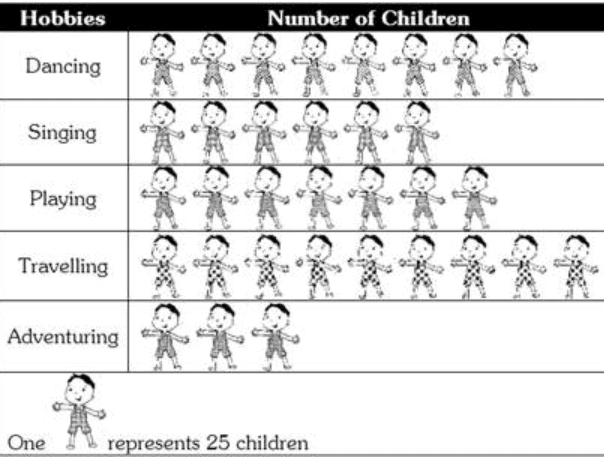Detailed Solution for Test: Data Handling - 1 - Question 1

3 × 25 = 75. There are 75 children whose hobby is adventuring.

Test: Data Handling - 1 - Question 2

### Find the number of children whose hobby is either travelling or adventuring?

Detailed Solution for Test: Data Handling - 1 - Question 2

Number of children whose hobby is travelling = 9 × 25 = 225 Number of children whose hobby is adventuring = 3×25=75 Total number of children whose hobby is either travelling or adventuring = 225 + 75 = 300.

Test: Data Handling - 1 - Question 3

### The marks obtained in 4 subjects in an examination are 200. If the average of marks obtained in 3 subjects is 48, then how many marks are obtained in fourth subject?

Detailed Solution for Test: Data Handling - 1 - Question 3

Total marks obtained in 4 subjects = 200 Average of 3 subjects = 48 Total sum of 3 subjects =  48 × 3 = 144 Fourth subject Marks = Total marks obtained - Total sum of 3 subjects.              = 200 - 155 = 56.

Test: Data Handling - 1 - Question 4

From the following graph determine which year faced maximum price rise in India in comparison to the preceding are: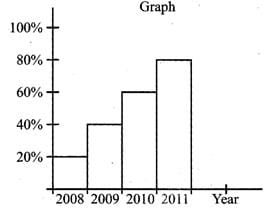Detailed Solution for Test: Data Handling - 1 - Question 4

In 2009, maximum price rise =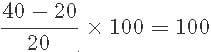In 2010, maximum price rise =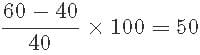In 2011, maximum price rise =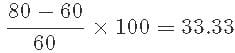So. Maximum price rise in India in comparison to the preceding year is 2009

Test: Data Handling - 1 - Question 5

If, ↑ represents 5 houses, then the number of houses represented by↑↑↑↑ are:

Detailed Solution for Test: Data Handling - 1 - Question 5

5 × 4

Test: Data Handling - 1 - Question 6

A graph drawn using pictures is called:

Detailed Solution for Test: Data Handling - 1 - Question 6

A graph that conveys its meaning using pictures is called pictograph.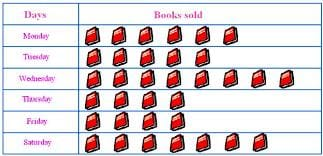Test: Data Handling - 1 - Question 7

A pictorial representation of data is called _______.

Detailed Solution for Test: Data Handling - 1 - Question 7

Among different type of data representations possible, the representation which consists of pictures i.e A pictorial representation of data is called pictograph.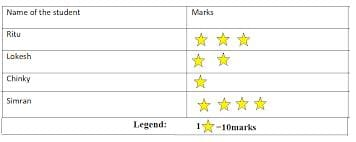Test: Data Handling - 1 - Question 8

The pictograph shows the distances of towns W, X and Y from town Z.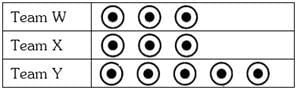Which of the following road maps shows the correct positions of towns W, X, Y and Z?

Detailed Solution for Test: Data Handling - 1 - Question 8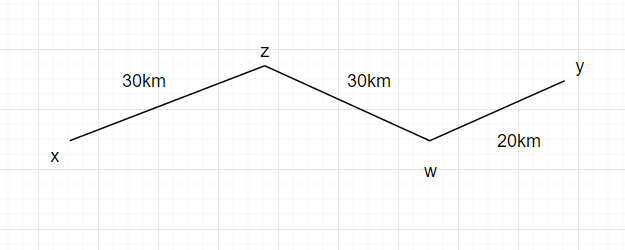Test: Data Handling - 1 - Question 9

The bar graph shows the grades obtained by a group of pupils in a test.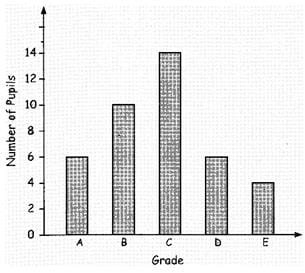If grade C is the passing mark, how many pupils passed the test?

Detailed Solution for Test: Data Handling - 1 - Question 9

Number of pupils passed by grade c are 14

Test: Data Handling - 1 - Question 10

The mode of a set of observations is the value which

Detailed Solution for Test: Data Handling - 1 - Question 10

By definition, mode is the value which occurs most frequently.

## Mathematics for Class 5

9 videos|54 docs|35 tests
 Use Code STAYHOME200 and get INR 200 additional OFF Use Coupon Code
Information about Test: Data Handling - 1 Page
In this test you can find the Exam questions for Test: Data Handling - 1 solved & explained in the simplest way possible. Besides giving Questions and answers for Test: Data Handling - 1, EduRev gives you an ample number of Online tests for practice

## Mathematics for Class 5

9 videos|54 docs|35 tests